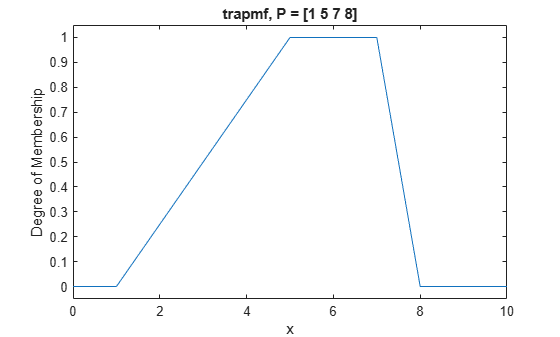# trapmf

Trapezoidal membership function

## Syntax

``y = trapmf(x,params)``

## Description

This function computes fuzzy membership values using a trapezoidal membership function. You can also compute this membership function using a `fismf` object. For more information, see fismf Object.

This membership function is related to the `trimf`, `linsmf`, and `linzmf` membership functions.

example

````y = trapmf(x,params)` returns fuzzy membership values computed using the following trapezoidal membership function:$f\left(x;a,b,c,d\right)=\mathrm{max}\left(\mathrm{min}\left(\frac{x-a}{b-a},1,\frac{d-x}{d-c}\right),0\right)$To define the membership function parameters, specify `params` as the vector [a b c d].Membership values are computed for each input value in `x`.```

## Examples

collapse all

Specify input values across the universe of discourse.

`x = 0:0.1:10;`

Evaluate membership function for the input values.

`y = trapmf(x,[1 5 7 8]);`

Plot the membership function.

```plot(x,y) title('trapmf, P = [1 5 7 8]') xlabel('x') ylabel('Degree of Membership') ylim([-0.05 1.05])```## Input Arguments

collapse all

Input values for which to compute membership values, specified as a scalar or vector.

Membership function parameters, specified as the vector [a b c d]. Parameters b and c define the shoulders of the membership function, and a and d define its feet.

The shape of the membership function depends on the relative values of b and c.

• When c is greater than b, the resulting membership function is trapezoidal.

• When b is equal to c, the resulting membership function is equivalent to a triangular membership function with parameters [a b d].

• When c is less than b, the resulting membership function is triangular with a maximum value less than 1.

## Output Arguments

collapse all

Membership value returned as a scalar or a vector. The dimensions of `y` match the dimensions of `x`. Each element of `y` is the membership value computed for the corresponding element of `x`.

## Alternative Functionality

### `fismf` Object

You can create and evaluate a `fismf` object that implements the `trapmf` membership function.

```mf = fismf("trapmf",P); Y = evalmf(mf,X);```

Here, `X`, `P`, and `Y` correspond to the `x`, `params`, and `y` arguments of `trapmf`, respectively.

## Version History

Introduced before R2006a

expand all